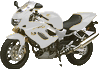OCR GCSE - Forces A

Page 1

 Speed, Acceleration, Graphs, Forces, Weight Choose the correct units for the following measurements : 1. Mass ? joules newtons kilograms m/s s N/m watts 2. Weight ? joules newtons kilograms m/s s N/m watts 3. Velocity ? joules newtons kilograms m/s s N/m watts 4. Which of the following are vector quantities? A). mass and velocity B). acceleration and force C). mass and displacement D). velocity and time ? A B C D This motorbike moves along a road at 25m/s.Calculate .. 5. The distance moved in 50s. A). 2 m B). 0.5 m C). 125 m D). 1.25 km ? A B C D 6. The time taken to travel 2 kilometres. A). 4 s B). 80 s C). 0.08 s D). 40 s ? A B C D 7. Which of these 4 distance (s) -time (t) graphs shows a horse moving at constant velocity?? A B C D 8. Which of these is the correct formula for acceleration ? A). change in velocity x time taken B). change in velocity / time taken C). velocity x time taken D). velocity / time taken ? A B C D 9. A train accelerates from 10 m/s to 50 m/s in 20 seconds. The acceleration is: A). 2 m/s2 B). 2.5 m/s2 C). 800 m/s2 D). 1000 m/s2 ? A B C D 10. "Whenever 2 objects interact, the forces between them are equal and opposite". This is A). Newton's First Law B). Newton's Second law C). Newton's Third law D). not one of Newton's Laws ? A B C D The velocity - time graph shows the journey of a bus as it moves away from a road junction. What quantity is shown by.....11. The gradient of line A ? acceleration deceleration distance travelled maximum velocity change in velocity average velocity 12. Line B ? acceleration deceleration distance travelled maximum velocity change in velocity average velocity 13. The area under the line. ? acceleration deceleration distance travelled maximum velocity change in velocity average velocity 14. The gradient of line C ? acceleration deceleration distance travelled maximum velocity change in velocity average velocity